E-Archive

Science Update

##### Key Factors on Cavitation Peening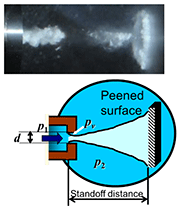Fig. 1 Schematic diagram of main parameter on cavitation peening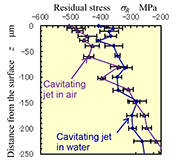Fig. 2 Introduction of compressive residual stress into stainless steel SUS316L by cavitating jet in air and water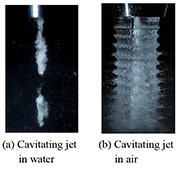Fig. 3 Aspects of cavitating jetFig. 4 Peening intensity as a function of normalized standoff distance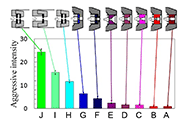Fig. 5 Nozzle geometry and the relative aggressive intensity of the cavitating jetFig. 6 Aspect of cavitating region ( = 0.03)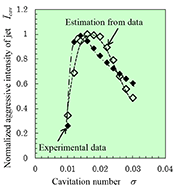Fig. 7 Effect of cavitation number on aggressive intensity of the cavitating jetFig. 8 Relative aggressive intensity of cavitating jet normalized by the value at p1 = 20 MPa at constant p2 = 0.1 MPa

Introduction

Cavitation normally causes severe damage in hydraulic machinery such as pumps and valves. However, cavitation impacts can be utilized for mechanical surface treatment in the same way of shot peening. A peening method using cavitation impacts is named as "cavitation peening" or "cavitation shotless peening", as shots are not required [1, 2].
In the case of cavitation peening, although a high-speed water jet is used to generate cavitation, cavitation peening is different from water jet peening in which water droplet impacts are used . Both peening methods can be classified by optimum standoff distance and cavitation number [2, 3]. As cavitation impacts are used in cavitation peening, enhancement and/or control of cavitation impact is very important.
In the present paper, key factors such as injection pressure p1, tank pressure p2, standoff distance s, nozzle diameter d on cavitation peening were discussed with experimental data (see Fig. 1). The white regions are cavitation bubbles in the upper part of Fig. 1. The estimation method of the aggressive intensity of the cavitating jet by key parameters was also proposed.

Type of Cavitating Jets

In the case of normal cavitation peening, cavitation is produced by injecting a high-speed water jet into a water-filled chamber: it is called a cavitating jet in water. Soyama realized a cavitating jet in air by injecting a high-speed water jet into a low-speed water jet that  was injected into air without a water-filled chamber.
From the viewpoint of introduction of compressive residual stress into metallic material, the cavitating jet in air can introduce large compressive residual stress near the surface. On the other hand, the cavitating jet in water can introduce compressive residual stress in deeper regions as shown in Fig. 2 . Namely, the distribution of compressive residual stress strongly depends on the type of cavitating jet, i.e., the cavitating jet in water and air. If a large compressive residual stress near the surface is required, the cavitating jet in air is suitable (see Fig. 3).

Standoff Distance

In the case of a cavitating jet, cavitation is initiated near the nozzle, developed and collapsed at a certain region. Thus, standoff distance, i.e., distance from nozzle to target, is one of the main parameters. Figure 4 reveals peening intensity as a function of standoff distance normalized throat diameter . In Fig. 4, the peening intensity is described by arc height. As shown in Fig. 4, the arc height has a peak at a certain standoff distance, i.e., optimum standoff distance. The relationship between the cavitation number and optimum standoff distance is an important factor to classify cavitation peening and water jet peening .
As shown in Fig. 4, the peening intensity using the cavitating jet at overly high injection pressure with small nozzle is smaller than that of relatively low injection pressure with a large nozzle. The reason of this tendency is discussed in  the section of "cavitation number".

Nozzle Geometry

As mentioned above, in the case of cavitation peening, cavitation is normally generated by injecting a high-speed water jet into a water-filled chamber or a low-speed water jet through a nozzle. Figure 5 illustrates the shapes of the nozzles and the relative aggressive intensity of the cavitating jet. Nozzles A, B, C, D and E are the nozzles for a conventional normal water jet. Nozzle F is the nozzle for the materials test to investigate the cavitation erosion resistance of the materials using a cavitating jet .
Nozzle G is an optimized nozzle considering nozzle outlet bore in order to enhance the aggressive intensity of the cavitating jet .
Nozzles F and G are developed for the cavitating jet. When the relative aggressive intensity is compared, the aggressive intensity of Nozzle G is about six times larger than that of Nozzle A. Namely, aggressive intensity of the cavitating jet through the nozzle for the cavitating jet is larger than that of the nozzle for the water jet.
As cavitation is initiated from cavitation nuclei, the cavitator, which generates cavitation nuclei, is installed as shown in nozzle H, where the aggressive intensity is enhanced about 1.8-fold compared with the nozzle without the cavitator . As mentioned above, the geometry of nozzle outlet bore, i.e., a kind of step, is very important to enhance aggressive intensity of the cavitating jet, as the vortex at nozzle exit is one of the key factors. Thus, another step, i.e., a guide pipe, was installed in Nozzle I, the aggressive intensity was   2.4-times larger than that of the nozzle without the guide pipe . When both  the cavitator and the guide pipe were installed, the aggressive intensity of the cavitating jet was about 4 times larger than that of the nozzle neither with the cavitator or with the guide pipe. Namely, the aggressive intensity of the cavitating jet of optimized nozzle with the cavitator and the guide pipe was about 24 times larger than that of a conventional nozzle for a water jet.

Cavitation Number

Cavitation number  which is defined by upstream pressure of nozzle, i.e., injection pressure p1, downstream pressure of nozzle, i.e., tank pressure p2 and vapor pressure of water pv as shown in Eq. (1), is most important parameter of cavitating flow.

"formula cannot be displayed online"        (1)

At the condition of small cavitation number, cavitating region is large, and the cavitating region is small at the large cavitation number. Namely, the cavitation number describes the size of the cavitating region. For example, at the constant cavitation number, the cavitating region is equal, even though the injection pressure was different, as shown in Fig. 6.
In the case of cavitating region and cavitating length, the cavitation number is a useful parameter. The optimum standoff distance sopt, where the aggressive intensity of the cavitating jet has a peak against the standoff distance, can be described by Eq. (2).

"formula cannot be displayed online"        (2)

Here, n is a constant.
The aggressive intensity has a peak at  ≈ 0.01 ~ 0.014 at constant injection pressure, as shown in Fig. 7. At the low cavitation number, cavitating region is developed; however, the surrounding pressure around the cavitation becomes low at constant injection pressure. Thus, the shrinking speed of cavitation bubble becomes slow and the shock wave at rebound is going to be weak. Namely, at too low a cavitation number, cavitation impact becomes weak. The effect of cavitation number on the aggressive intensity of the cavitating jet can be described by Eq. (3), considering pseudo vapor pressure pv’ and the shift of cavitation number s .

"formula cannot be displayed online"        (3)

In Fig. 7, the estimated value of the aggressive intensity of the cavitating jet from experimental data is also revealed.

Nozzle Throat Diameter

In the case of cavitation erosion, which is caused by cavitation impacts, scale effect has been reported. The cavitation erosion rate is increasing with the size of hydraulic machinery. Normally, the power law is reported, and the exponent is scattered. In the case of cavitating jet, the aggressive intensity of the jet is increased with nozzle throat diameter, and the power law such as Eq. (4) was proposed .

"formula cannot be displayed online"        (4)

Here, nd is exponent of power law on nozzle throat diameter. The nd is about 2 and it is changing with  .

Injection Pressure

In the case of cavitation erosion, erosion rate is also increasing with flow speed. The flow speed of the cavitating jet is proportional to the square root of injection pressure. Thus, the power law on injection pressure such as Eq. (5) was also proposed .

"formula cannot be displayed online"        (5)

Here, np is exponent of power law on injection pressure. The np is from 1 to 2 and it is changing with  .

Downstream Pressure (Tank Pressure)

As shown in the section of "cavitation number", downstream pressure of nozzle, i.e., the tank pressure is one of the  parameters of cavitation number. The aggressive intensity of the jet has a peak at certain downstream pressure, which is changing with the injection pressure. On the other hand, the cavitation number at which the aggressive intensity has a peak is constant, even though the injection pressure is changed. Thus, it can be concluded that the cavitation number is the more important factor.

Experimental Formula to Estimate Aggressive Intensity of Cavitation

As the aggressive intensity of the cavitating jet Icav is affected by nozzle geometry, cavitation number , nozzle throat diameter d and the injection pressure p1, the experimental formula to estimate the aggressive intensity of the jet was proposed as shown in Eq. (6).

"formula cannot be displayed online"        (6)

Here, Kn is shape function and it depends on the geometry of the nozzle. Relative aggressive intensity is revealed in Fig. 5. The nd and np are the exponents of the power laws, which are described in Eqs. (4) and (5); these are obtained  experimentally . The f() was the influence function on cavitation number  and it can be expressed by Eq. (3). The ref means the value at the reference condition. When the aggressive intensity at certain conditions was measured, the aggressive intensity of the jet at the other condition can be estimated by using Eq. (6).
In order to prove the applicability of Eq. (6), aggressive intensity of the jet obtained experimentally is plotted by □, and the estimated value is described by the dotted line in Fig. 8.

Conclusions

In order to obtain better peening effect by cavitation peening effectively, parameters on cavitation peening were discussed. The distribution of introduced compressive residual stress depends on the type of cavitating jet, i.e., the cavitating jet in water and air. In the case of cavitating jet in water, the relative aggressive intensity of the jet can be estimated by nozzle geometry, cavitation number, nozzle throat diameter and injection pressure using Eq. (6).

Acknowledgement

This work was partly supported by JSPS KAKENHI Grant Number 17H03138. The author thanks Mr. M. Mikami, technician, Tohoku University for his help with the experiment.

References

 H. Soyama, "Surface Mechanics Design of Metallic Materials on Mechanical Surface Treatments" Mechanical Engineering Reviews, vol. 2, paper No. 14-00192, no. 1, pp. 1-20, 2015.
 H. Soyama, "Key Factors and Applications of Cavitation Peening," International Journal of Peening Science and Technology, vol. 1, no. 1, pp. in press, 2017.
 H. Soyama, "Criterion on Mechanical Surface Treatment Using a Submerged Water Jet between Cavitation Peening and Water Jet Peening," Jet Flow Engineering, vol. 31, No. 2, no. 2, pp. 1-8, 2015.
 H. Soyama, K. Nishizawa, and M. Mikami, "Comparison of Abilities of Various Cavitating Jets," 9th Pacific Rim International Conference on Water Jetting Technology, pp. 133-137, 2009.
 G134-95, "Standard Test Method for Erosion of Solid Materials by a Cavitating Liquid Jet," ASTM standard, vol. 03.02, pp. 1-14, 2006.
 H. Soyama, "Enhancing the Aggressive Intensity of a Cavitating Jet by Means of the Nozzle Outlet Geometry," Journal of Fluids Engineering, vol. 133, paper No. 101301, no. 10, pp. 1-11, 2011.
 H. Soyama, "Enhancing the Aggressive Intensity of a Cavitating Jet by Introducing a Cavitator and a Guide Pipe," Journal of Fluid Science and Technology, vol. 9, paper No. 13-00238, no. 1, pp. 1-12, 2014.
 H. Soyama, "Power Law of Injection Pressure and Nozzle Diameter on Aggressive Intensity of a Cavitating Jet," Proceedings 21st International Conference on Water Jetting, pp. 343-354, 2012.

Department of Finemechanics
Tohoku University
6-6-01 Aoba, Aramaki, Aoba-ku, Sendai
980-8579, Japan
E-mail: soyama@mm.mech.tohoku.ac.jp